# Standard Template Library STL Standard Template Library Recall

• Slides: 20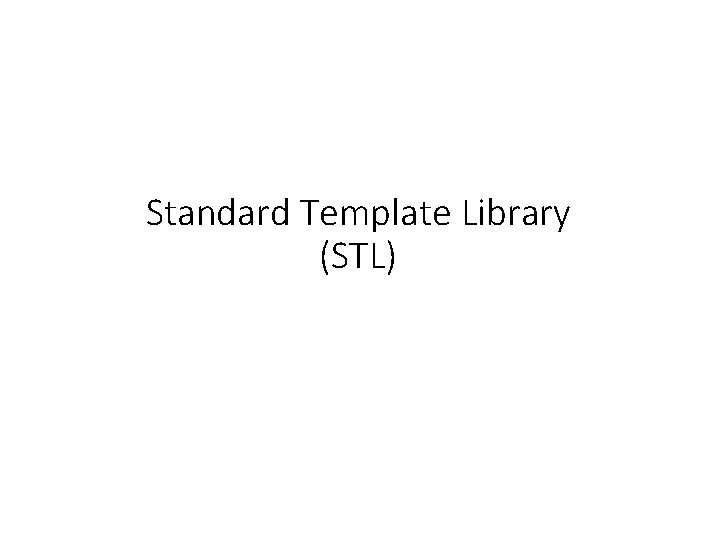Standard Template Library (STL)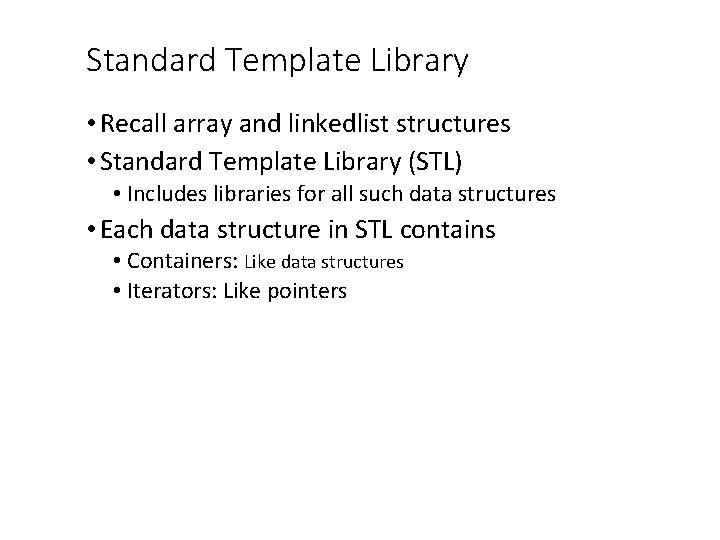Standard Template Library • Recall array and linkedlist structures • Standard Template Library (STL) • Includes libraries for all such data structures • Each data structure in STL contains • Containers: Like data structures • Iterators: Like pointers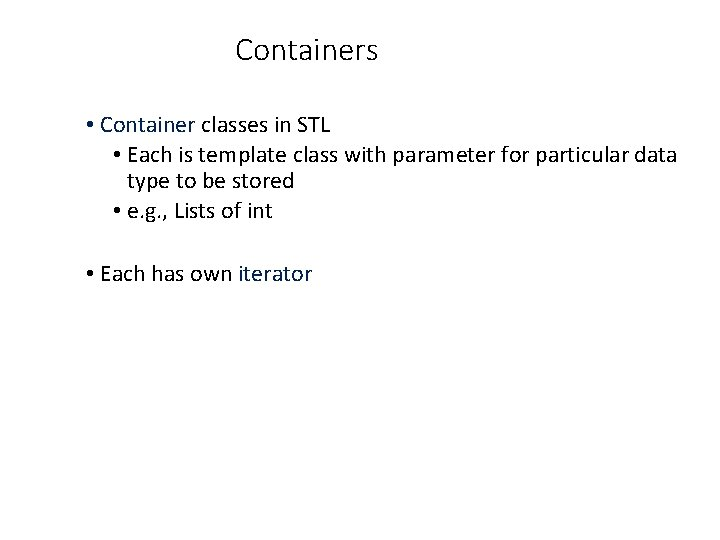Containers • Container classes in STL • Each is template class with parameter for particular data type to be stored • e. g. , Lists of int • Each has own iterator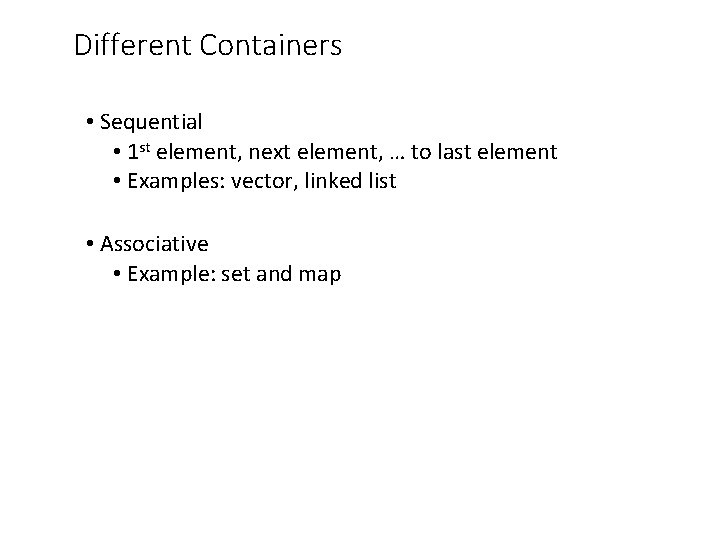Different Containers • Sequential • 1 st element, next element, … to last element • Examples: vector, linked list • Associative • Example: set and map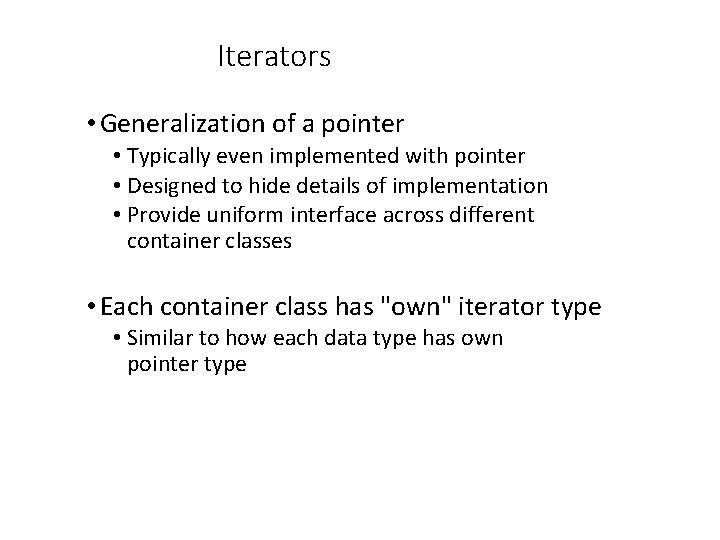Iterators • Generalization of a pointer • Typically even implemented with pointer • Designed to hide details of implementation • Provide uniform interface across different container classes • Each container class has "own" iterator type • Similar to how each data type has own pointer type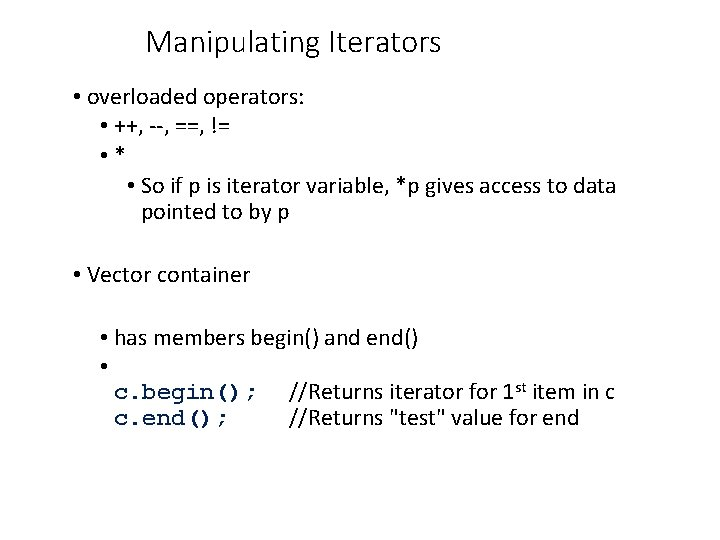Manipulating Iterators • overloaded operators: • ++, --, ==, != • * • So if p is iterator variable, *p gives access to data pointed to by p • Vector container • has members begin() and end() • c. begin(); //Returns iterator for 1 st item in c c. end(); //Returns "test" value for end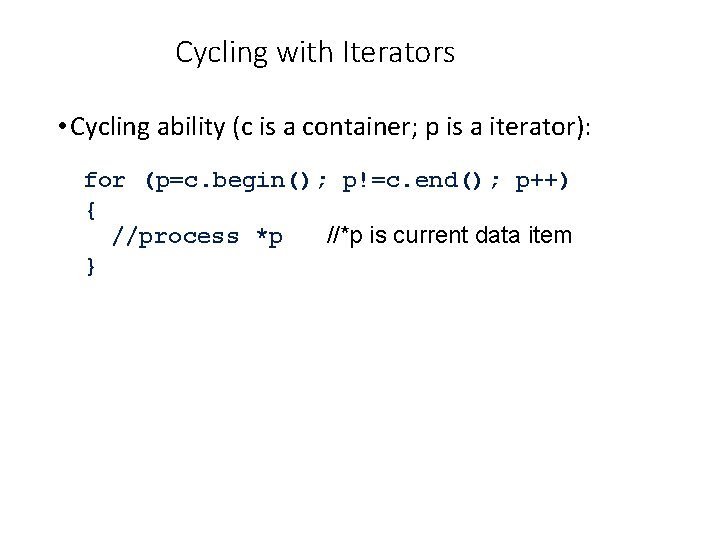Cycling with Iterators • Cycling ability (c is a container; p is a iterator): for (p=c. begin(); p!=c. end(); p++) { //process *p //*p is current data item }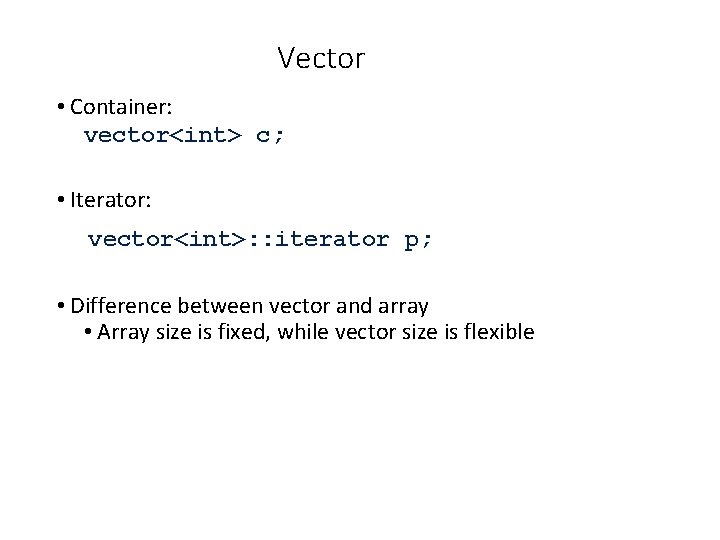Vector • Container: vector<int> c; • Iterator: vector<int>: : iterator p; • Difference between vector and array • Array size is fixed, while vector size is flexibleIterators Used with a Vector #include <iostream> #include <vector> int main( ){ vector<int> c; vector<int>: : iterator p; for (int i = 1; i <= 4; i++) c. push_back(i); // initialize vector cout << "Here is what is in the container: n"; for (p = c. begin( ); p != c. end( ); p++) cout << *p << " "; cout << endl;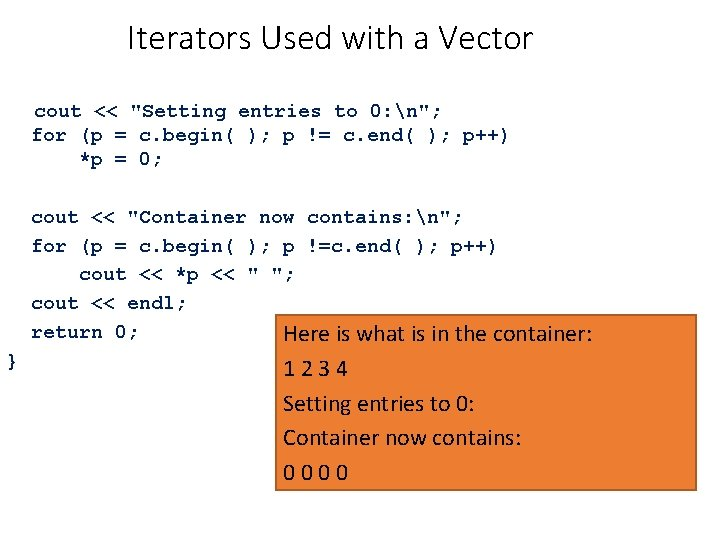Iterators Used with a Vector cout << "Setting entries to 0: n"; for (p = c. begin( ); p != c. end( ); p++) *p = 0; cout << "Container now contains: n"; for (p = c. begin( ); p !=c. end( ); p++) cout << *p << " "; cout << endl; return 0; Here is what is in the container: } 1234 Setting entries to 0: Container now contains: 0000Random Access of vector iterators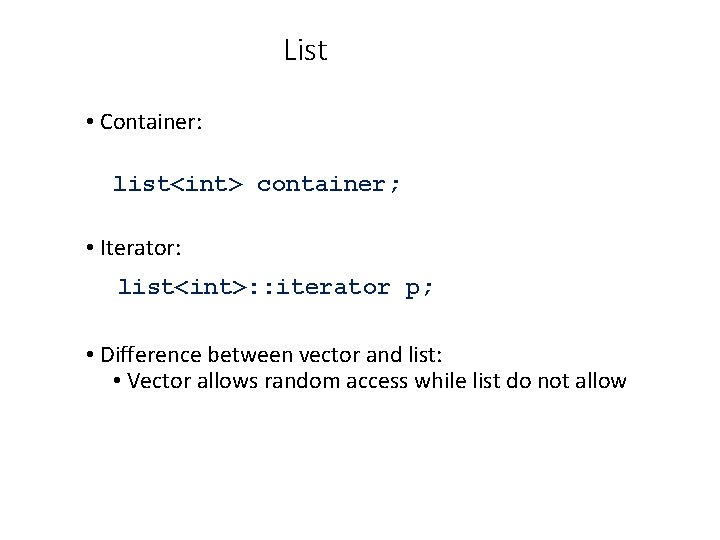List • Container: list<int> container; • Iterator: list<int>: : iterator p; • Difference between vector and list: • Vector allows random access while list do not allowTwo Kinds of Lists STL list is a double link list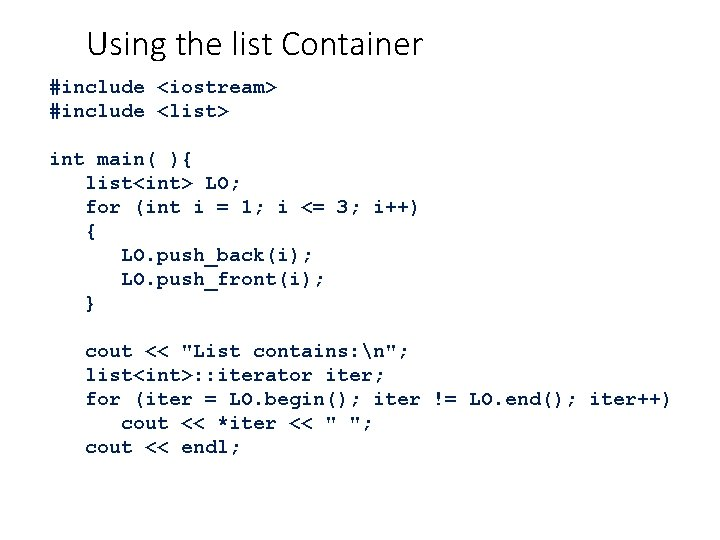Using the list Container #include <iostream> #include <list> int main( ){ list<int> LO; for (int i = 1; i <= 3; i++) { LO. push_back(i); LO. push_front(i); } cout << "List contains: n"; list<int>: : iterator iter; for (iter = LO. begin(); iter != LO. end(); iter++) cout << *iter << " "; cout << endl;Using the list Container cout << "Setting all entries to 0: n"; for (iter = LO. begin(); iter != LO. end(); iter++) *iter = 0; cout << "List now contains: n"; for (iter = LO. begin(); iter != LO. end(); iter++) cout << *iter << " "; cout << endl; return 0; } List contains: 3 2 1 1 2 3 Setting all entries to 0: List now contains: 0 0 0Set Container • Stores elements without repetition • 1 st insertion places element in set • Each element value is its own key • Capabilities: • Add elements • Delete elements • Ask if element is in set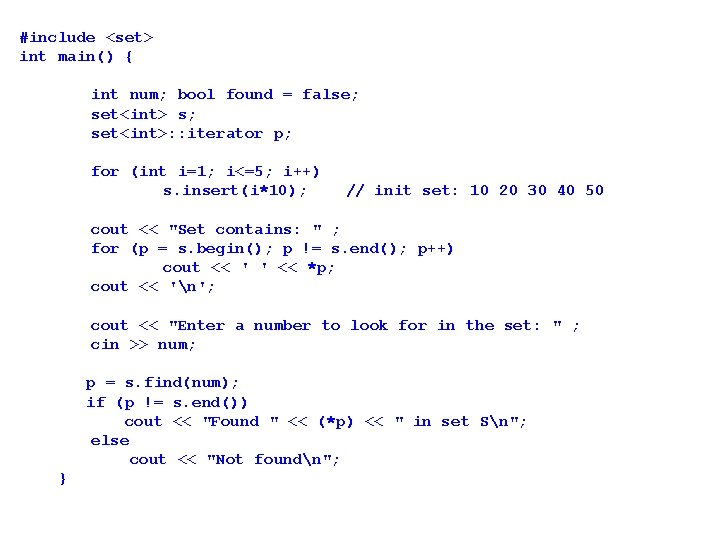#include <set> int main() { int num; bool found = false; set<int> s; set<int>: : iterator p; for (int i=1; i<=5; i++) s. insert(i*10); // init set: 10 20 30 40 50 cout << "Set contains: " ; for (p = s. begin(); p != s. end(); p++) cout << ' ' << *p; cout << 'n'; cout << "Enter a number to look for in the set: " ; cin >> num; p = s. find(num); if (p != s. end()) cout << "Found " << (*p) << " in set Sn"; else cout << "Not foundn"; }cout << "n. Enter a number to erase from set: " ; cin >> num; if (s. find(num)!= s. end()) { // don't erase if not in set s. erase(num); cout << "Erasing " << num << " from set. . . n"; } else cout << num << " is not in set and can't be erasedn"; cout << "Set contains: " ; for (p = s. begin(); p != s. end(); p++) cout << " " << *p; cout << "nn"; }Set contains: 10 20 30 40 50 Enter a number to look for in the set: 20 Found 20 in set S Enter a number to erase from set: 35 35 is not in set and can't be erased Set contains: 10 20 30 40 50 : : Set contains: 10 20 30 40 50 Enter a number to look for in the set: 25 Not found Enter a number to erase from set: 30 Erasing 30 from set. . . Set contains: 10 20 40 50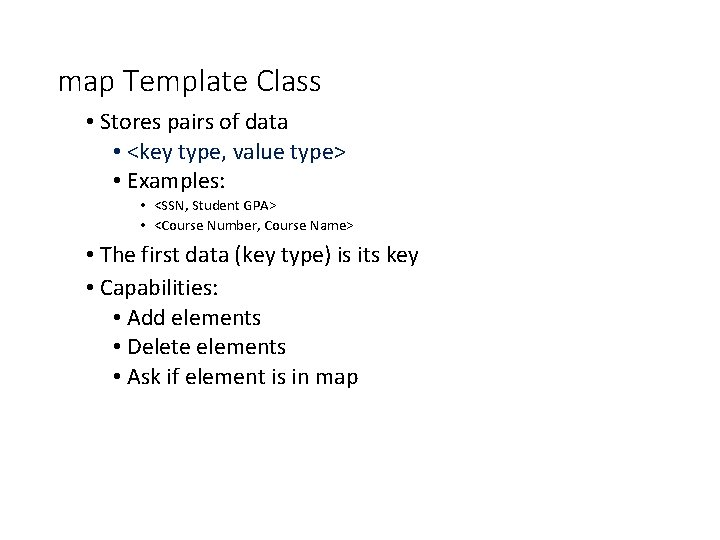map Template Class • Stores pairs of data • <key type, value type> • Examples: • <SSN, Student GPA> • <Course Number, Course Name> • The first data (key type) is its key • Capabilities: • Add elements • Delete elements • Ask if element is in map